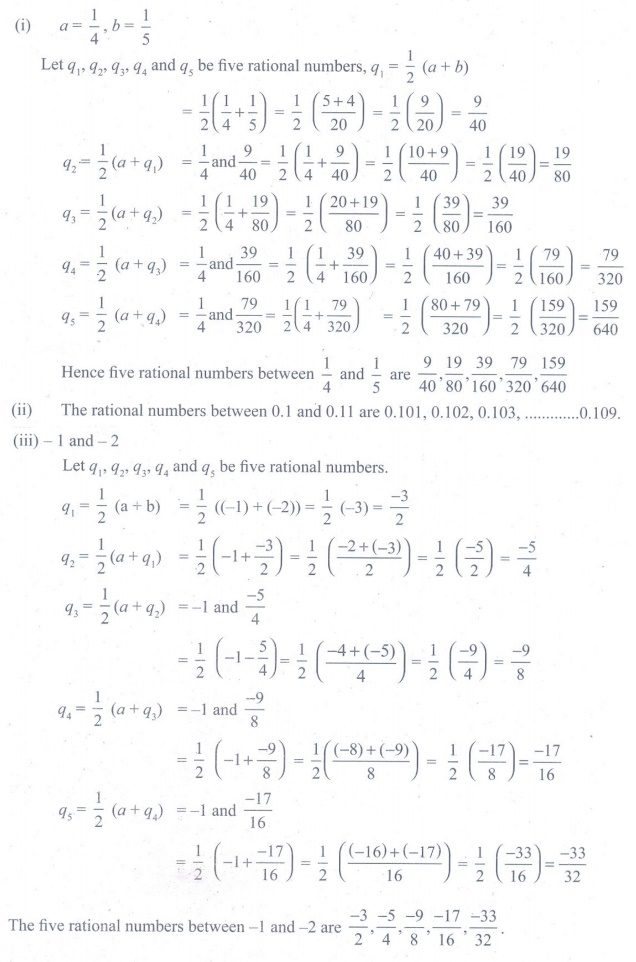Home | | Maths 9th std | Exercise 2.1: Rational Numbers

# Exercise 2.1: Rational Numbers

Maths : Real Numbers: Book Back, Exercise, Example Numerical Question with Answers, Solution : Numerical Problems with Answers, Solution : Exercise 2.1: Rational Numbers

Exercise 2.1

1. Which arrow best shows the position of 11/3 on the number line?2. Find any three rational numbers between -7/11 and 2/11 .3. Find any five rational numbers between (i) 1/4 and 1/5 (ii) 0.1 and 0.11 (iii) –1 and –2Tags : Numerical Problems with Answers, Solution | Real Numbers | Maths , 9th Maths : UNIT 2 : Real Numbers
Study Material, Lecturing Notes, Assignment, Reference, Wiki description explanation, brief detail
9th Maths : UNIT 2 : Real Numbers : Exercise 2.1: Rational Numbers | Numerical Problems with Answers, Solution | Real Numbers | Maths